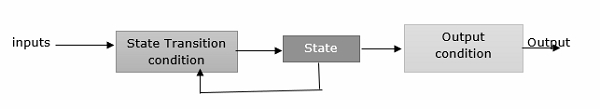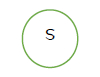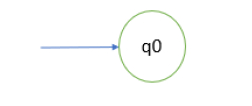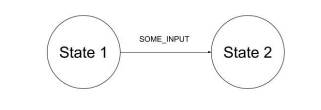# What is a finite state machine in TOC?

A finite state machine has a set of states and two functions called the next-state and output function.

• The set of states correspond to all the possible combinations of the internal storage. If there are n bits of storage, there are 2n possible states.
• The next state function is a combinational logic function that, given the inputs and the current state, determines the next state of the system.

The diagram given below explains the functioning of a finite state machine in TOC.The output function generates a set of outputs from the current state and the inputs.

## Types

The two types of finite state machines are −

• Moore machine − In Moore machine, the output only depends on the current state.
• Mealy machine − In Mealy machine, the output depends on both the current state and the current input.

We mostly deal with the Moore machine. These two types are equivalent in capabilities.

A Finite State Machine consists of the following −

• K states: S = {s1, s2, ... ,sk}, s1 is initial state
• N inputs: I = {h, i2, ... ,in}
• M outputs: O = {o1, o2, . ,om}
• Next-state function T(S, I): This is used for mapping each current state and input to the next state.
• Output Function P(S): This function specifies the output.

## Components

The components which exists in a finite state machine are explained below −

State − The states are usually drawn with circles and only one state can be active at a time.

It is represented as follows −Initial State − It is the starting point of our system. Initial states are usually drawn with an arrow pointed to state, as shown below −Final state − It is a subset of known states that indicates whether the input we processed is valid or not. Accepting states are usually drawn as a double circle as shown below −Transitions − The machine moves from one state to another and is indicated as transition. These are drawn as two states connected with a line, as shown below −